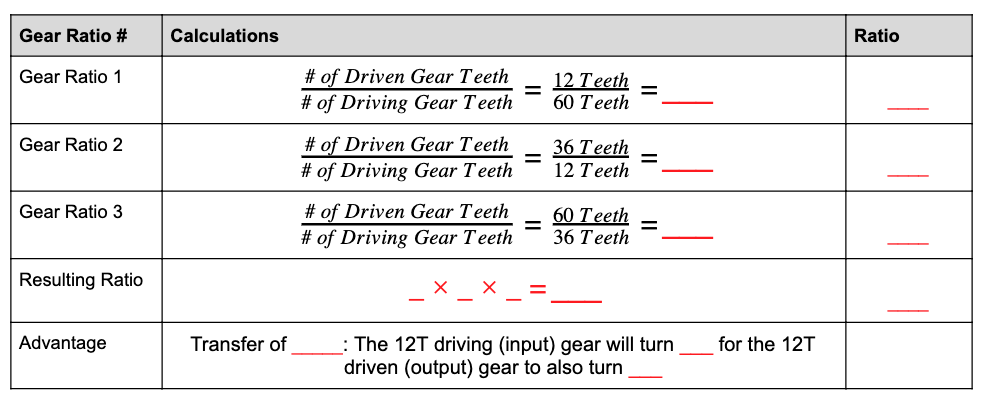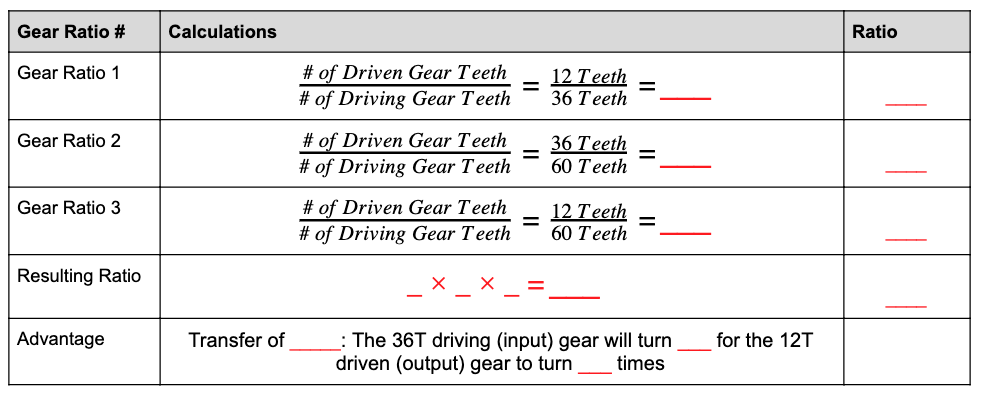STEMLabs IQ
Lab 12 - M.A.D. Box

# Calculating Three Gear Ratios

Now that you have calculated a compound gear ratio from two gear ratios, we will now calculate a compound gear ratio from three gear ratios!

You will work in groups of four to calculate gear ratios and determine the resulting mechanical advantage.

### Calculation 2

Fill in the missing calculations from the Gear Ratio table. Keep in mind, each person should be calculating according to their role.• Role 1: Calculate the Gear Ratio 1 row of the above table. Show all work in your engineering notebook.

• Role 2: Calculate the Gear Ratio 2 row of the above table. Show all work in your engineering notebook.

• Role 3: Calculate the Gear Ratio 3 row of the above table. Show all work in your engineering notebook.

• Role 4: Calculate the Resulting Ratio row of the above table. Check the calculations from Gear Ratio 1, 2, and 3 before calculating the final compound gear ratio. Show all work in your engineering notebook.

• All Roles: Calculate the Advantage row of the above table. Show all work in your engineering notebook.

### Calculation 3

Fill in the missing calculations from the Gear Ratio table. Keep in mind, each person should be calculating according to their role.• Role 1: Calculate the Gear Ratio 1 row of the above table. Show all work in your engineering notebook.

• Role 2: Calculate the Gear Ratio 2 row of the above table. Show all work in your engineering notebook.

• Role 3: Calculate the Gear Ratio 3 row of the above table. Show all work in your engineering notebook.

• Role 4: Calculate the Resulting Ratio row of the above table. Check the calculations from Gear Ratio 1, 2, and 3 before calculating the final compound gear ratio. Show all work in your engineering notebook.

• All Roles: Calculate the Advantage row of the above table. Show all work in your engineering notebook.